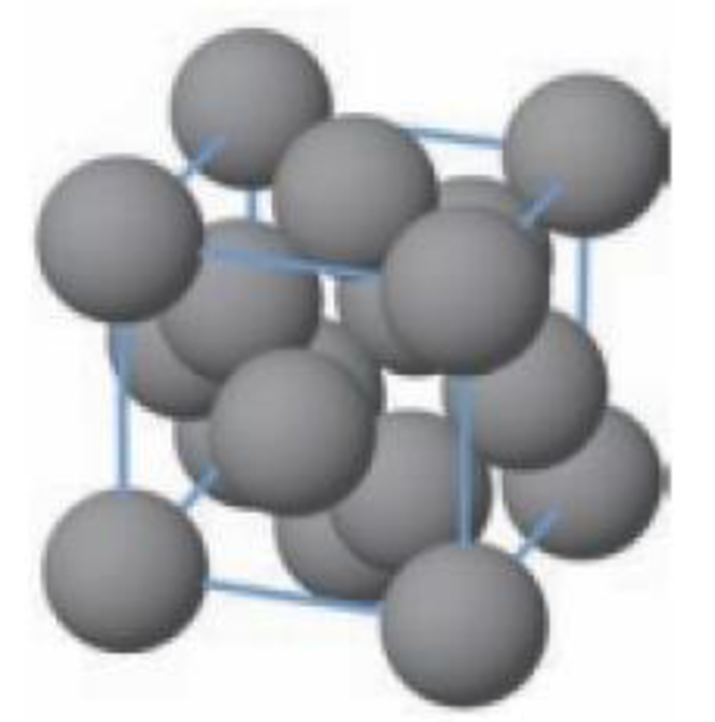Chapter 12, Problem 29PS

Chapter
Section
Textbook Problem

A diamond unit cell is shown here.Unit cell of diamond (a) How many carbon atoms are in one unit cell? (b) The unit cell can be considered as a cubic unit cell of C atoms with other C atoms in holes in the lattice. What type of unit cell is this (pc, bcc, fcc)? In what holes are other C atoms located, octahedral or tetrahedral holes?

(a)

Interpretation Introduction

Interpretation:

Number of carbon atoms has to be identified.

Concept introduction:

The face-centered cubic system:

It has lattice points on the faces of the cube, that each gives exactly one half contributions, in addition to the corner lattice points, giving a total of 4 lattice points per unit cell

Explanation

There are eight carbon atoms per unit cell. Because, Number of atoms per unit is given below,

It has 8 atoms in the corner, 6 atom in the face, 4 internal carbon atoms in the body.

Number of atoms per unit=(8corneratoms×1/8+6

(b)

Interpretation Introduction

Interpretation:

The type of the unit cell and arrangement of carbon atoms has to be identified.

Concept introduction:

The face-centered cubic system:

It has lattice points on the faces of the cube, that each gives exactly one half contribution, in addition to the corner lattice points, giving a total of 4 lattice points per unit cell

Still sussing out bartleby?

Check out a sample textbook solution.

See a sample solution

The Solution to Your Study Problems

Bartleby provides explanations to thousands of textbook problems written by our experts, many with advanced degrees!

Get Started

Taking supplements of fish oil is recommended for those who don't like fish. T F

Nutrition: Concepts and Controversies - Standalone book (MindTap Course List)

What does density describe?

An Introduction to Physical Science

How does recombinant DNA differ from normal DNA?

General, Organic, and Biological Chemistry